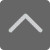## 爱智康资讯站

### 课程咨询: 400-810-2680

• 全国课程在线咨询
• 咨询热线：4000-121-121
• 登录手机官网

• 关注微信公众号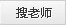# 数学丨高三2020-2021学年下期中考后知识点梳理

2021-04-23 21:54:06 　来源：百度文库

## 学而思1对1 帮助孩子解决知识难点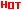*2470人已预约，首课体验不满意，可全额退费。

― ― 报名课程可获得 ― ―点击免费领取→学而思1对1 学习资料库！

高三数学知识点总结

命题的“否定”与命题的“否命题”是两个不同的概念，命题p的否定是否定命题所作的判断，而“否命题”是对“若p，则q”形式的命题而言，既要否定条件也要否定结论。

集合中的元素具有确定性、无序性、互异性，集合元素的三性中互异性对解题的影响较大，特别是带有字母参数的集合，实际上就隐含着对字母参数的一些要求。

判断函数的奇偶性，首先要考虑函数的定义域，一个函数具备奇偶性的必要条件是这个函数的定义域关于原点对称，如果不具备这个条件，函数一定是非奇非偶函数。

如果函数y=f(x)在区间[a，b]上的图像是一条连续的曲线，并且有f(a)f(b)<0，那么，函数y=f(x)在区间(a，b)内有零点，但f(a)f(b)>0时，不能否定函数y=f(x)在(a，b)内有零点。函数的零点有“变号零点”和“不变号零点”，对于“不变号零点”函数的零点定理是“无能为力”的，在解决函数的零点问题时要注意这个问题。

在研究函数问题时要时时刻刻想到“函数的图像”，学会从函数图像上去分析问题、寻找解决问题的方法。对于函数的几个不同的单调递增(减)区间，切忌使用并集，只要指明这几个区间是该函数的单调递增(减)区间即可。

对于函数y=Asin(ωx+φ)的单调性，当ω>0时，由于内层函数u=ωx+φ是单调递增的，所以该函数的单调性和y=sin x的单调性相同，故可完全按照函数y=sin x的单调区间解决;但当ω<0时，内层函数u=ωx+φ是单调递减的，此时该函数的单调性和函数y=sinx的单调性相反，就不能再按照函数y=sinx的单调性解决，一般是根据三角函数的奇偶性将内层函数的系数变为正数后再加以解决。对于带有少有值的三角函数应该根据图像，从直观上进行判断。

解题时要全面考虑问题。数学试题中往往隐含着一些容易被考生所忽视的因素，能不能在解题时把这些因素考虑到，是解题成功的关键，如当a·b<0时，a与b的夹角不一定为钝角，要注意θ=π的情况。

零向量是向量中较特殊的向量，规定零向量的长度为0，其方向是任意的，零向量与任意向量都共线。它在向量中的位置正如实数中0的位置一样，但有了它容易引起一些混淆，稍微考虑不到就会出错，考生应给予足够的重视。

等差数列的前n项和在公差不为零时是关于n的常数项为零的二次函数;一般地，有结论“若数列{an}的前n项和Sn=an2+bn+c(a，b，c∈R)，则数列{an}为等差数列的充要条件是c=0”;在等差数列中，Sm，S2m-Sm，S3m-S2m(m∈N*)是等差数列。

在数列问题中，数列的通项an与其前n项和Sn之间存在下列关系：an=S1，n=1，Sn-Sn-1，n≥2。这个关系对任意数列都是成立的，但要注意的是这个关系式是分段的，在n=1和n≥2时这个关系式具有完全不同的表现形式，这也是解题中经常出错的一个地方，在使用这个关系式时要牢牢记住其“分段”的特点。

高三数学背诵的公式

乘法与因式分 a2-b2=(a+b)(a-b) a3+b3=(a+b)(a2-ab+b2) a3-b3=(a-b(a2+ab+b2)

三角不等式 |a+b|≤|a|+|b| |a-b|≤|a|+|b| |a|≤b<=>-b≤a≤b

|a-b|≥|a|-|b| -|a|≤a≤|a|

一元二次方程的解 -b+√(b2-4ac)/2a -b-√(b2-4ac)/2a

根与系数的关系 X1+X2=-b/a X1*X2=c/a 注：韦达定理

判别式

b2-4ac=0 注：方程有两个相等的实根

b2-4ac>0 注：方程有两个不等的实根

b2-4ac<0 注：方程没有实根，有共轭复数根

三角函数公式

两角和公式

sin(A+B)=sinAcosB+cosAsinB sin(A-B)=sinAcosB-sinBcosA

cos(A+B)=cosAcosB-sinAsinB cos(A-B)=cosAcosB+sinAsinB

tan(A+B)=(tanA+tanB)/(1-tanAtanB) tan(A-B)=(tanA-tanB)/(1+tanAtanB)

ctg(A+B)=(ctgActgB-1)/(ctgB+ctgA) ctg(A-B)=(ctgActgB+1)/(ctgB-ctgA)

倍角公式

tan2A=2tanA/(1-tan2A) ctg2A=(ctg2A-1)/2ctga

cos2a=cos2a-sin2a=2cos2a-1=1-2sin2a

半角公式

sin(A/2)=√((1-cosA)/2) sin(A/2)=-√((1-cosA)/2)

cos(A/2)=√((1+cosA)/2) cos(A/2)=-√((1+cosA)/2)

tan(A/2)=√((1-cosA)/((1+cosA)) tan(A/2)=-√((1-cosA)/((1+cosA))

ctg(A/2)=√((1+cosA)/((1-cosA)) ctg(A/2)=-√((1+cosA)/((1-cosA))

和差化积

2sinAcosB=sin(A+B)+sin(A-B) 2cosAsinB=sin(A+B)-sin(A-B)

2cosAcosB=cos(A+B)-sin(A-B) -2sinAsinB=cos(A+B)-cos(A-B)

sinA+sinB=2sin((A+B)/2)cos((A-B)/2 cosA+cosB=2cos((A+B)/2)sin((A-B)/2)

tanA+tanB=sin(A+B)/cosAcosB tanA-tanB=sin(A-B)/cosAcosB

ctgA+ctgBsin(A+B)/sinAsinB -ctgA+ctgBsin(A+B)/sinAsinB

学好高中数学的方法

认真听课适当做笔记,不放过任何联想小结的机会是读好书的关键。上课的内容有难有易，不能因为容易而轻视它，也不能因为困难而害怕它。容易的问题思维强度小，但所提供的思维空间却很大，可以把自己的方法与老师的方法进行整合，对相关的问题进行小结，对问题的发展进行预测，为后面更难的问题积累充足的思维惯性。

弄清概念、性质和基本方法是每个学科学习的先进步也是较重要的一步，如果概念没有弄清就去解题是没有不碰壁的。正确理解概念再做题目就比较容易了，通过题目的演算反过来还可以进一步理解概念与性质。

在小学初中时复习靠老师，到了高中复习要靠自己。因为在高中的课程多，内容广，所以在课堂上不可能经常反复。一节课内容一个星期之内不复习就有可能变得陌生，较好是三天内复习一次。

数学丨高三2020-2021学年下期中考后知识点梳理就给大家分享到这里，想了解相关课程的同学，请拨打学而思1对1免费咨询电话：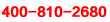另外学而思学科老师还给大家整理了一份《小、初、高学习资料包》。点击免费领取：《学而思1对1 学习资料库！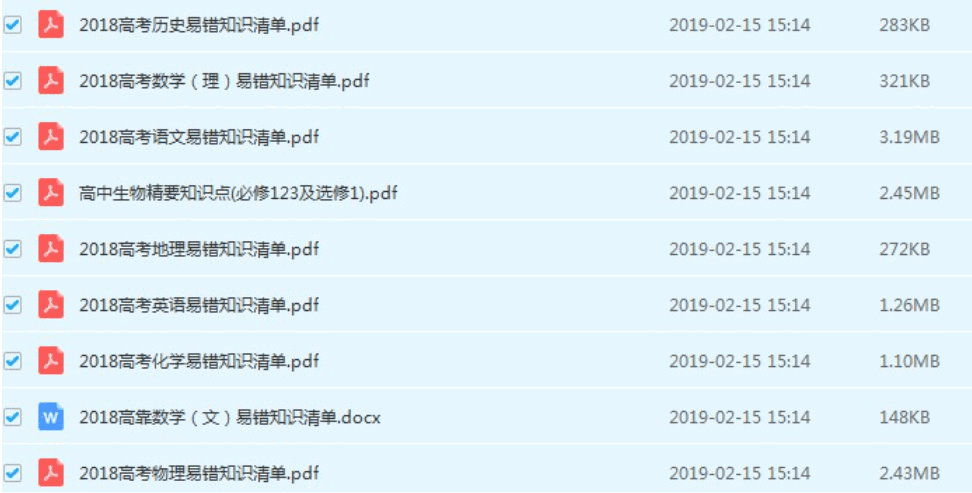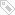标签：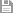保存　｜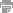打印　｜关闭